Скачать презентацию Applied Mathematical Sciences Vol 7 2013 no 6

fee56d3b49c9858332f9c41fc4b6c952.ppt

• Количество слайдов: 16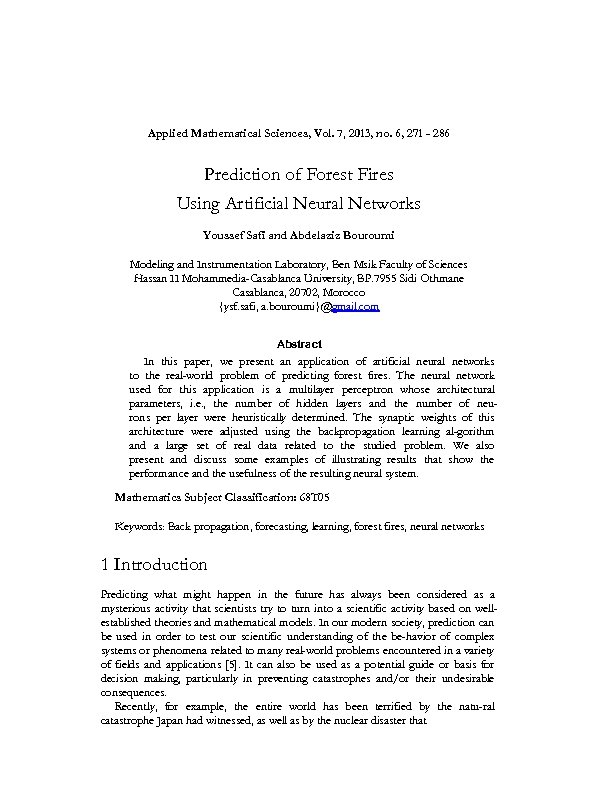Applied Mathematical Sciences, Vol. 7, 2013, no. 6, 271 - 286 Prediction of Forest Fires Using Artificial Neural Networks Youssef Safi and Abdelaziz Bouroumi Modeling and Instrumentation Laboratory, Ben Msik Faculty of Sciences Hassan II Mohammedia-Casablanca University, BP. 7955 Sidi Othmane Casablanca, 20702, Morocco {ysf. safi, a. bouroumi}@gmail. com Abstract In this paper, we present an application of artificial neural networks to the real-world problem of predicting forest fires. The neural network used for this application is a multilayer perceptron whose architectural parameters, i. e. , the number of hidden layers and the number of neurons per layer were heuristically determined. The synaptic weights of this architecture were adjusted using the backpropagation learning al-gorithm and a large set of real data related to the studied problem. We also present and discuss some examples of illustrating results that show the performance and the usefulness of the resulting neural system. Mathematics Subject Classification: 68 T 05 Keywords: Back propagation, forecasting, learning, forest fires, neural networks 1 Introduction Predicting what might happen in the future has always been considered as a mysterious activity that scientists try to turn into a scientific activity based on wellestablished theories and mathematical models. In our modern society, prediction can be used in order to test our scientific understanding of the be-havior of complex systems or phenomena related to many real-world problems encountered in a variety of fields and applications . It can also be used as a potential guide or basis for decision making, particularly in preventing catastrophes and/or their undesirable consequences. Recently, for example, the entire world has been terrified by the natu-ral catastrophe Japan had witnessed, as well as by the nuclear disaster that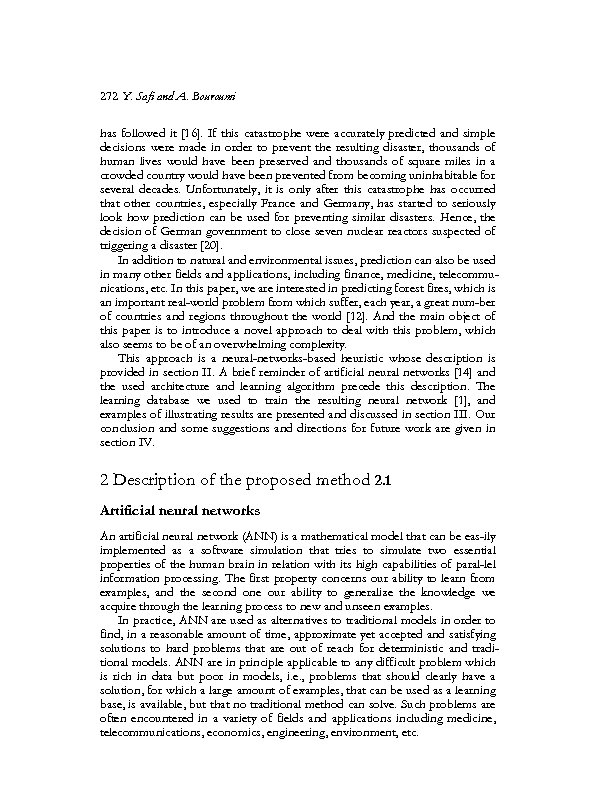272 Y. Safi and A. Bouroumi has followed it . If this catastrophe were accurately predicted and simple decisions were made in order to prevent the resulting disaster, thousands of human lives would have been preserved and thousands of square miles in a crowded country would have been prevented from becoming uninhabitable for several decades. Unfortunately, it is only after this catastrophe has occurred that other countries, especially France and Germany, has started to seriously look how prediction can be used for preventing similar disasters. Hence, the decision of German government to close seven nuclear reactors suspected of triggering a disaster . In addition to natural and environmental issues, prediction can also be used in many other fields and applications, including finance, medicine, telecommunications, etc. In this paper, we are interested in predicting forest fires, which is an important real-world problem from which suffer, each year, a great num-ber of countries and regions throughout the world . And the main object of this paper is to introduce a novel approach to deal with this problem, which also seems to be of an overwhelming complexity. This approach is a neural-networks-based heuristic whose description is provided in section II. A brief reminder of artificial neural networks  and the used architecture and learning algorithm precede this description. The learning database we used to train the resulting neural network , and examples of illustrating results are presented and discussed in section III. Our conclusion and some suggestions and directions for future work are given in section IV. 2 Description of the proposed method 2. 1 Artificial neural networks An artificial neural network (ANN) is a mathematical model that can be eas-ily implemented as a software simulation that tries to simulate two essential properties of the human brain in relation with its high capabilities of paral-lel information processing. The first property concerns our ability to learn from examples, and the second one our ability to generalize the knowledge we acquire through the learning process to new and unseen examples. In practice, ANN are used as alternatives to traditional models in order to find, in a reasonable amount of time, approximate yet accepted and satisfying solutions to hard problems that are out of reach for deterministic and traditional models. ANN are in principle applicable to any difficult problem which is rich in data but poor in models, i. e. , problems that should clearly have a solution, for which a large amount of examples, that can be used as a learning base, is available, but that no traditional method can solve. Such problems are often encountered in a variety of fields and applications including medicine, telecommunications, economics, engineering, environment, etc.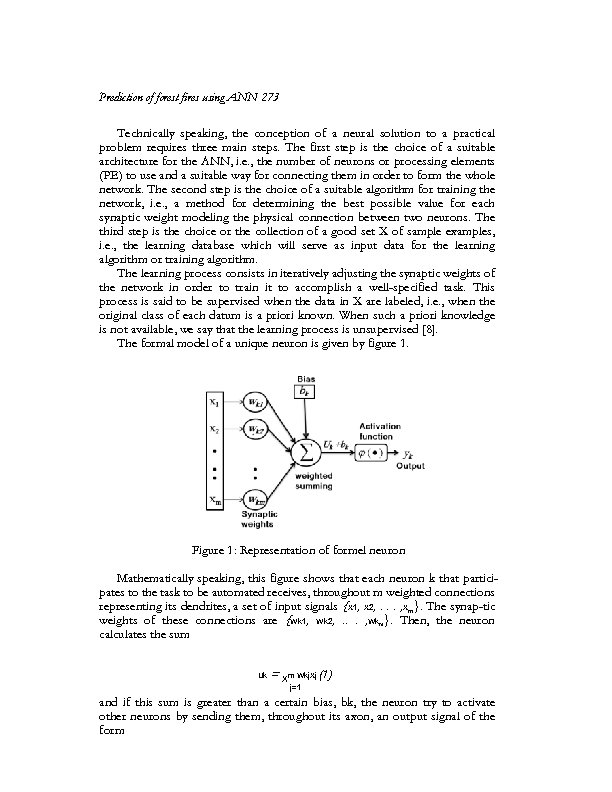Prediction of forest fires using ANN 273 Technically speaking, the conception of a neural solution to a practical problem requires three main steps. The first step is the choice of a suitable architecture for the ANN, i. e. , the number of neurons or processing elements (PE) to use and a suitable way for connecting them in order to form the whole network. The second step is the choice of a suitable algorithm for training the network, i. e. , a method for determining the best possible value for each synaptic weight modeling the physical connection between two neurons. The third step is the choice or the collection of a good set X of sample examples, i. e. , the learning database which will serve as input data for the learning algorithm or training algorithm. The learning process consists in iteratively adjusting the synaptic weights of the network in order to train it to accomplish a well-specified task. This process is said to be supervised when the data in X are labeled, i. e. , when the original class of each datum is a priori known. When such a priori knowledge is not available, we say that the learning process is unsupervised . The formal model of a unique neuron is given by figure 1. Figure 1: Representation of formel neuron Mathematically speaking, this figure shows that each neuron k that participates to the task to be automated receives, throughout m weighted connections representing its dendrites, a set of input signals {x 1, x 2, . . . , xm}. The synap-tic weights of these connections are {wk 1, wk 2, . . . , wkm}. Then, the neuron calculates the sum uk = Xm wkjxj (1) j=1 and if this sum is greater than a certain bias, bk, the neuron try to activate other neurons by sending them, throughout its axon, an output signal of the form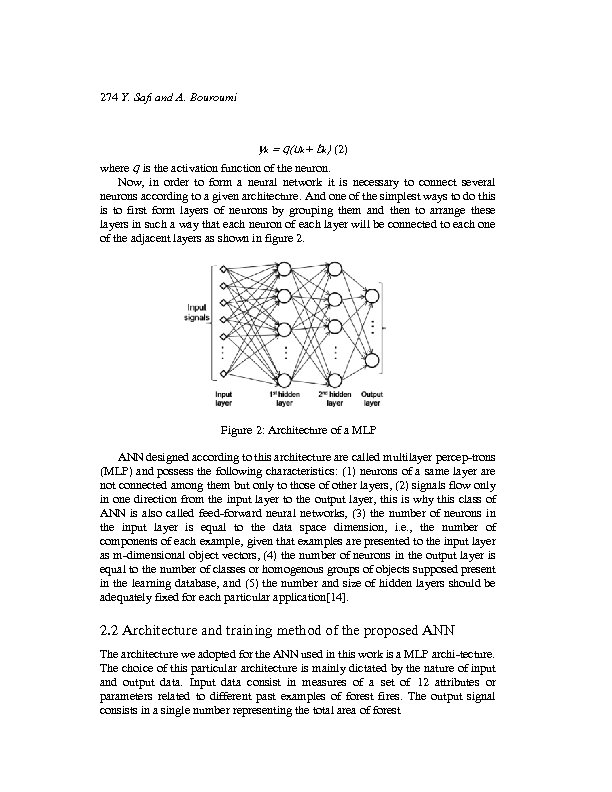274 Y. Safi and A. Bouroumi yk = q(uk + bk) (2) where q is the activation function of the neuron. Now, in order to form a neural network it is necessary to connect several neurons according to a given architecture. And one of the simplest ways to do this is to first form layers of neurons by grouping them and then to arrange these layers in such a way that each neuron of each layer will be connected to each one of the adjacent layers as shown in figure 2. Figure 2: Architecture of a MLP ANN designed according to this architecture are called multilayer percep-trons (MLP) and possess the following characteristics: (1) neurons of a same layer are not connected among them but only to those of other layers, (2) signals flow only in one direction from the input layer to the output layer, this is why this class of ANN is also called feed-forward neural networks, (3) the number of neurons in the input layer is equal to the data space dimension, i. e. , the number of components of each example, given that examples are presented to the input layer as m-dimensional object vectors, (4) the number of neurons in the output layer is equal to the number of classes or homogenous groups of objects supposed present in the learning database, and (5) the number and size of hidden layers should be adequately fixed for each particular application. 2. 2 Architecture and training method of the proposed ANN The architecture we adopted for the ANN used in this work is a MLP archi-tecture. The choice of this particular architecture is mainly dictated by the nature of input and output data. Input data consist in measures of a set of 12 attributes or parameters related to different past examples of forest fires. The output signal consists in a single number representing the total area of forest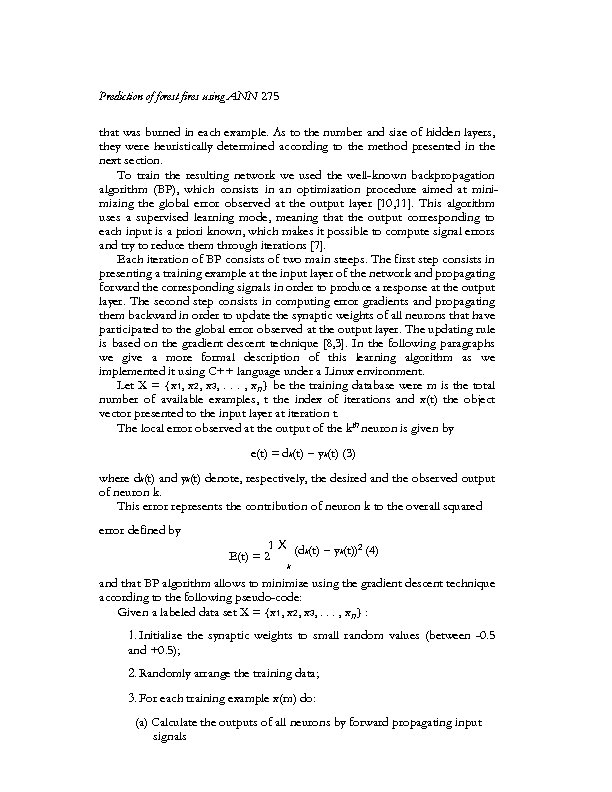Prediction of forest fires using ANN 275 that was burned in each example. As to the number and size of hidden layers, they were heuristically determined according to the method presented in the next section. To train the resulting network we used the well-known backpropagation algorithm (BP), which consists in an optimization procedure aimed at minimizing the global error observed at the output layer [10, 11]. This algorithm uses a supervised learning mode, meaning that the output corresponding to each input is a priori known, which makes it possible to compute signal errors and try to reduce them through iterations . Each iteration of BP consists of two main steeps. The first step consists in presenting a training example at the input layer of the network and propagating forward the corresponding signals in order to produce a response at the output layer. The second step consists in computing error gradients and propagating them backward in order to update the synaptic weights of all neurons that have participated to the global error observed at the output layer. The updating rule is based on the gradient descent technique [8, 3]. In the following paragraphs we give a more formal description of this learning algorithm as we implemented it using C++ language under a Linux environment. Let X = {x 1, x 2, x 3, . . . , xn} be the training database were m is the total number of available examples, t the index of iterations and x(t) the object vector presented to the input layer at iteration t. The local error observed at the output of the kth neuron is given by e(t) = dk(t) − yk(t) (3) where dk(t) and yk(t) denote, respectively, the desired and the observed output of neuron k. This error represents the contribution of neuron k to the overall squared error defined by 1 X (dk(t) − yk(t))2 (4) E(t) = 2 k and that BP algorithm allows to minimize using the gradient descent technique according to the following pseudo-code: Given a labeled data set X = {x 1, x 2, x 3, . . . , xn} : 1. Initialize the synaptic weights to small random values (between -0. 5 and +0. 5); 2. Randomly arrange the training data; 3. For each training example x(m) do: (a) Calculate the outputs of all neurons by forward propagating input signals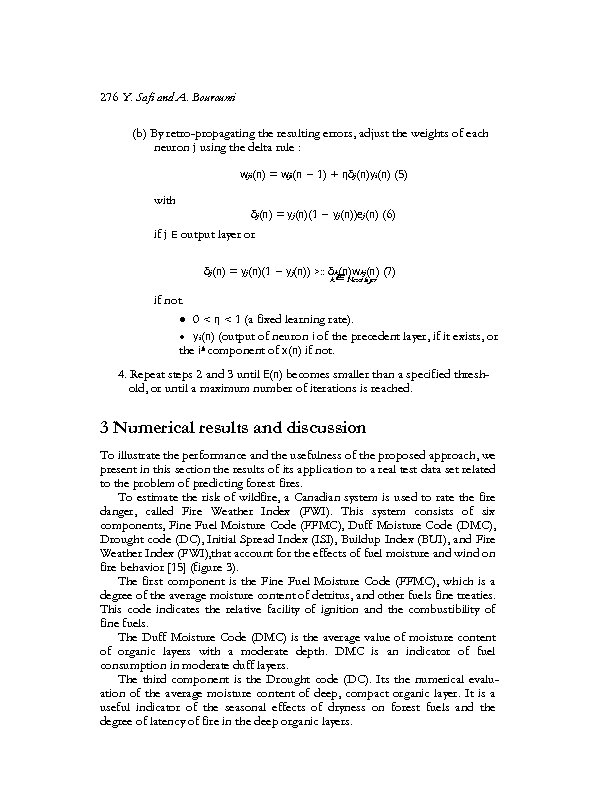276 Y. Safi and A. Bouroumi (b) By retro-propagating the resulting errors, adjust the weights of each neuron j using the delta rule : wji(n) = wji(n − 1) + ηδj(n)yi(n) (5) with δj(n) = yj(n)(1 − yj(n))ej(n) (6) if j E output layer or δj(n) = yj(n)(1 − yj(n)) >: : δk(n)wkj(n) (7) k∈ Next layer if not. · 0 < η < 1 (a fixed learning rate). · yi(n) (output of neuron i of the precedent layer, if it exists, or the ith component of x(n) if not. 4. Repeat steps 2 and 3 until E(n) becomes smaller than a specified threshold, or until a maximum number of iterations is reached. 3 Numerical results and discussion To illustrate the performance and the usefulness of the proposed approach, we present in this section the results of its application to a real test data set related to the problem of predicting forest fires. To estimate the risk of wildfire, a Canadian system is used to rate the fire danger, called Fire Weather Index (FWI). This system consists of six components, Fine Fuel Moisture Code (FFMC), Duff Moisture Code (DMC), Drought code (DC), Initial Spread Index (ISI), Buildup Index (BUI), and Fire Weather Index (FWI), that account for the effects of fuel moisture and wind on fire behavior  (figure 3). The first component is the Fine Fuel Moisture Code (FFMC), which is a degree of the average moisture content of detritus, and other fuels fine treaties. This code indicates the relative facility of ignition and the combustibility of fine fuels. The Duff Moisture Code (DMC) is the average value of moisture content of organic layers with a moderate depth. DMC is an indicator of fuel consumption in moderate duff layers. The third component is the Drought code (DC). Its the numerical evaluation of the average moisture content of deep, compact organic layer. It is a useful indicator of the seasonal effects of dryness on forest fuels and the degree of latency of fire in the deep organic layers.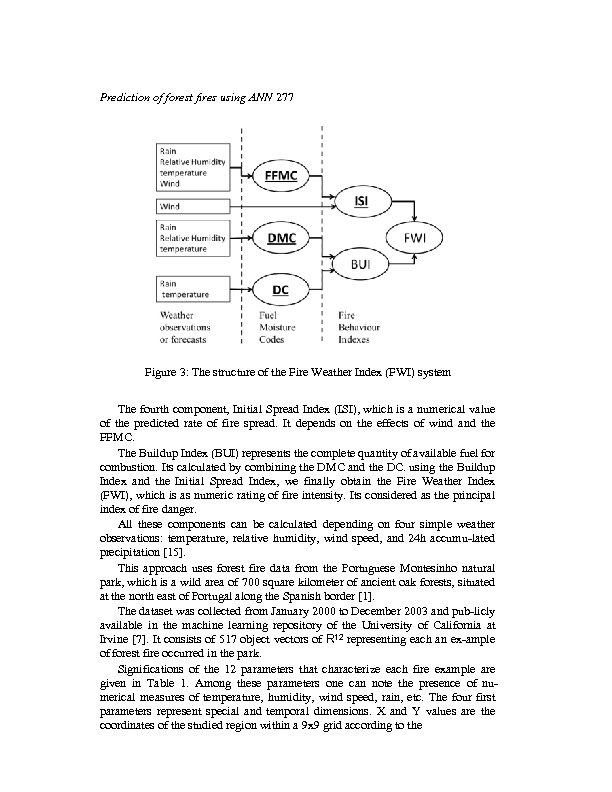Prediction of forest fires using ANN 277 Figure 3: The structure of the Fire Weather Index (FWI) system The fourth component, Initial Spread Index (ISI), which is a numerical value of the predicted rate of fire spread. It depends on the effects of wind and the FFMC. The Buildup Index (BUI) represents the complete quantity of available fuel for combustion. Its calculated by combining the DMC and the DC. using the Buildup Index and the Initial Spread Index, we finally obtain the Fire Weather Index (FWI), which is as numeric rating of fire intensity. Its considered as the principal index of fire danger. All these components can be calculated depending on four simple weather observations: temperature, relative humidity, wind speed, and 24 h accumu-lated precipitation . This approach uses forest fire data from the Portuguese Montesinho natural park, which is a wild area of 700 square kilometer of ancient oak forests, situated at the north east of Portugal along the Spanish border . The dataset was collected from January 2000 to December 2003 and pub-licly available in the machine learning repository of the University of California at Irvine . It consists of 517 object vectors of R 12 representing each an ex-ample of forest fire occurred in the park. Significations of the 12 parameters that characterize each fire example are given in Table 1. Among these parameters one can note the presence of numerical measures of temperature, humidity, wind speed, rain, etc. The four first parameters represent special and temporal dimensions. X and Y values are the coordinates of the studied region within a 9 x 9 grid according to the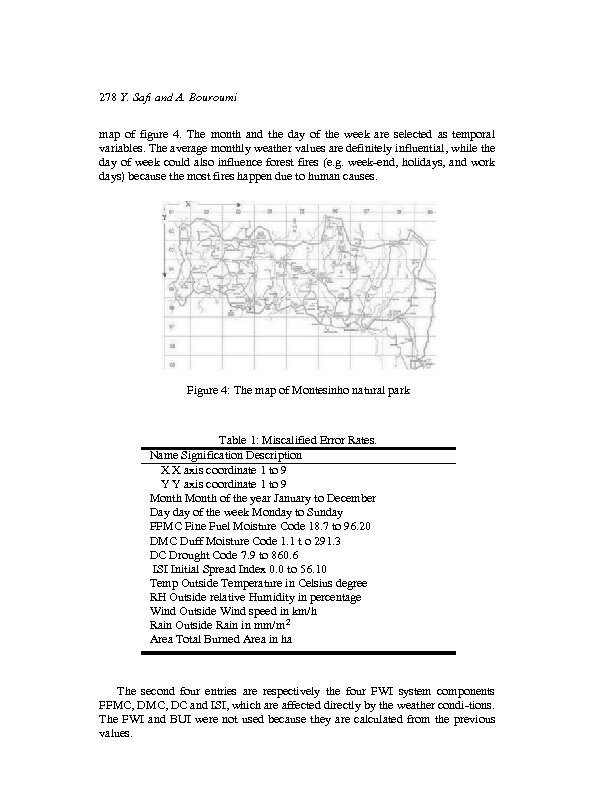278 Y. Safi and A. Bouroumi map of figure 4. The month and the day of the week are selected as temporal variables. The average monthly weather values are definitely influential, while the day of week could also influence forest fires (e. g. week-end, holidays, and work days) because the most fires happen due to human causes. Figure 4: The map of Montesinho natural park Table 1: Miscalified Error Rates. Name Signification Description X X axis coordinate 1 to 9 Y Y axis coordinate 1 to 9 Month of the year January to December Day day of the week Monday to Sunday FFMC Fine Fuel Moisture Code 18. 7 to 96. 20 DMC Duff Moisture Code 1. 1 t o 291. 3 DC Drought Code 7. 9 to 860. 6 ISI Initial Spread Index 0. 0 to 56. 10 Temp Outside Temperature in Celsius degree RH Outside relative Humidity in percentage Wind Outside Wind speed in km/h Rain Outside Rain in mm/m 2 Area Total Burned Area in ha The second four entries are respectively the four FWI system components FFMC, DC and ISI, which are affected directly by the weather condi-tions. The FWI and BUI were not used because they are calculated from the previous values.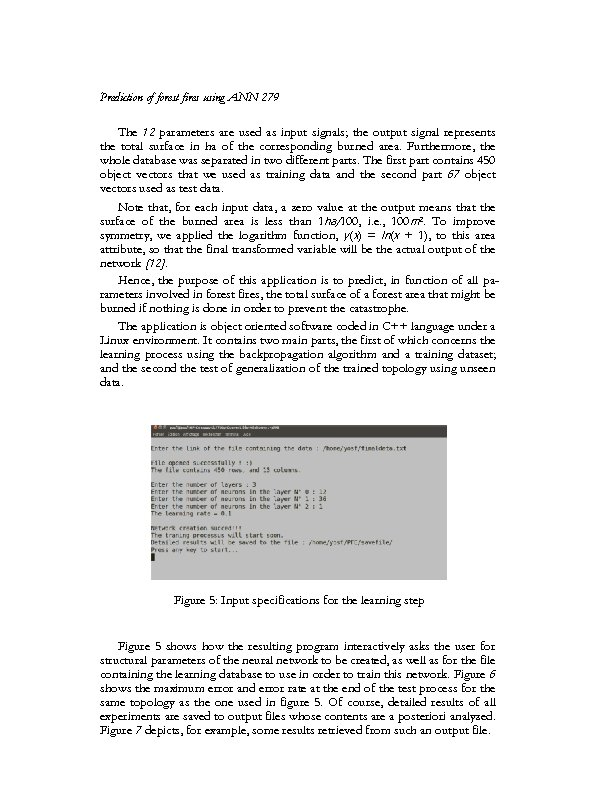Prediction of forest fires using ANN 279 The 12 parameters are used as input signals; the output signal represents the total surface in ha of the corresponding burned area. Furthermore, the whole database was separated in two different parts. The first part contains 450 object vectors that we used as training data and the second part 67 object vectors used as test data. Note that, for each input data, a zero value at the output means that the surface of the burned area is less than 1 ha/100, i. e. , 100 m 2. To improve symmetry, we applied the logarithm function, y(x) = ln(x + 1), to this area attribute, so that the final transformed variable will be the actual output of the network . Hence, the purpose of this application is to predict, in function of all parameters involved in forest fires, the total surface of a forest area that might be burned if nothing is done in order to prevent the catastrophe. The application is object oriented software coded in C++ language under a Linux environment. It contains two main parts, the first of which concerns the learning process using the backpropagation algorithm and a training dataset; and the second the test of generalization of the trained topology using unseen data. Figure 5: Input specifications for the learning step Figure 5 shows how the resulting program interactively asks the user for structural parameters of the neural network to be created, as well as for the file containing the learning database to use in order to train this network. Figure 6 shows the maximum error and error rate at the end of the test process for the same topology as the one used in figure 5. Of course, detailed results of all experiments are saved to output files whose contents are a posteriori analyzed. Figure 7 depicts, for example, some results retrieved from such an output file.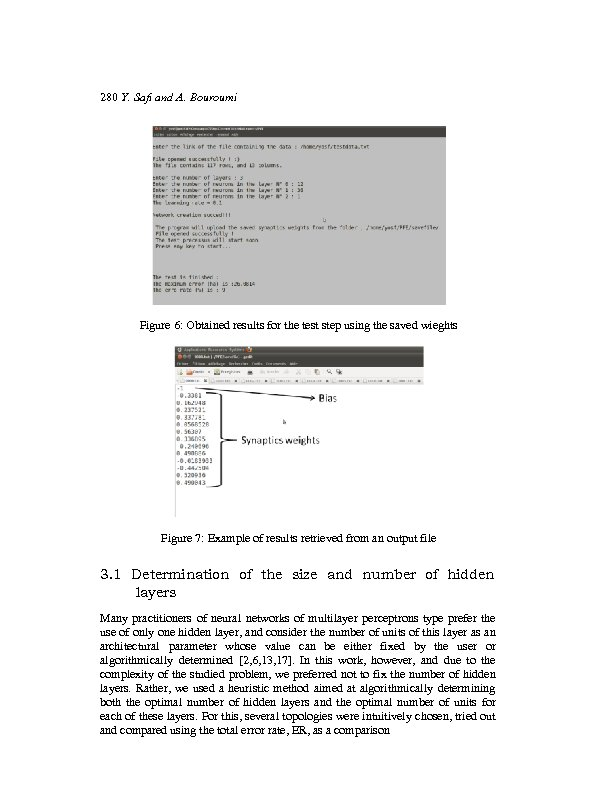280 Y. Safi and A. Bouroumi Figure 6: Obtained results for the test step using the saved wieghts Figure 7: Example of results retrieved from an output file 3. 1 Determination of the size and number of hidden layers Many practitioners of neural networks of multilayer perceptrons type prefer the use of only one hidden layer, and consider the number of units of this layer as an architectural parameter whose value can be either fixed by the user or algorithmically determined [2, 6, 13, 17]. In this work, however, and due to the complexity of the studied problem, we preferred not to fix the number of hidden layers. Rather, we used a heuristic method aimed at algorithmically determining both the optimal number of hidden layers and the optimal number of units for each of these layers. For this, several topologies were intuitively chosen, tried out and compared using the total error rate, ER, as a comparison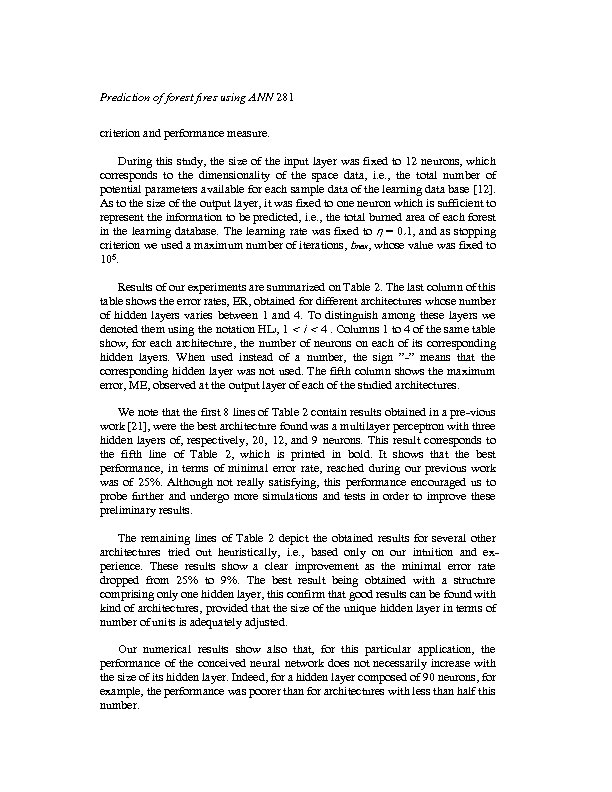Prediction of forest fires using ANN 281 criterion and performance measure. During this study, the size of the input layer was fixed to 12 neurons, which corresponds to the dimensionality of the space data, i. e. , the total number of potential parameters available for each sample data of the learning data base . As to the size of the output layer, it was fixed to one neuron which is sufficient to represent the information to be predicted, i. e. , the total burned area of each forest in the learning database. The learning rate was fixed to η = 0. 1, and as stopping criterion we used a maximum number of iterations, tmax, whose value was fixed to 105. Results of our experiments are summarized on Table 2. The last column of this table shows the error rates, ER, obtained for different architectures whose number of hidden layers varies between 1 and 4. To distinguish among these layers we denoted them using the notation HLi, 1 < i < 4. Columns 1 to 4 of the same table show, for each architecture, the number of neurons on each of its corresponding hidden layers. When used instead of a number, the sign ”-” means that the corresponding hidden layer was not used. The fifth column shows the maximum error, ME, observed at the output layer of each of the studied architectures. We note that the first 8 lines of Table 2 contain results obtained in a pre-vious work , were the best architecture found was a multilayer perceptron with three hidden layers of, respectively, 20, 12, and 9 neurons. This result corresponds to the fifth line of Table 2, which is printed in bold. It shows that the best performance, in terms of minimal error rate, reached during our previous work was of 25%. Although not really satisfying, this performance encouraged us to probe further and undergo more simulations and tests in order to improve these preliminary results. The remaining lines of Table 2 depict the obtained results for several other architectures tried out heuristically, i. e. , based only on our intuition and experience. These results show a clear improvement as the minimal error rate dropped from 25% to 9%. The best result being obtained with a structure comprising only one hidden layer, this confirm that good results can be found with kind of architectures, provided that the size of the unique hidden layer in terms of number of units is adequately adjusted. Our numerical results show also that, for this particular application, the performance of the conceived neural network does not necessarily increase with the size of its hidden layer. Indeed, for a hidden layer composed of 90 neurons, for example, the performance was poorer than for architectures with less than half this number.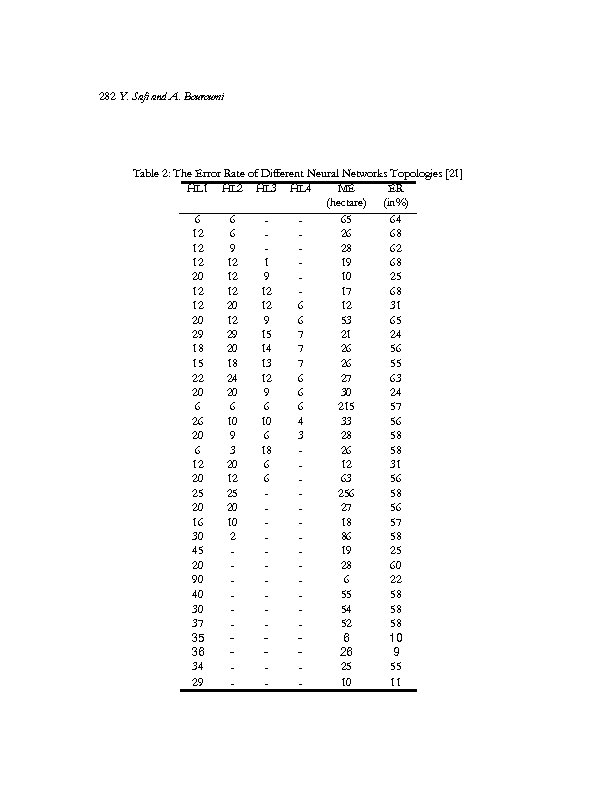282 Y. Safi and A. Bouroumi Table 2: The Error Rate of Different Neural Networks Topologies  HL 1 HL 2 HL 3 HL 4 ME ER (hectare) (in%) 6 6 65 64 12 6 26 68 12 9 28 62 12 12 1 19 68 20 12 9 10 25 12 12 12 17 68 12 20 12 6 12 31 20 12 9 6 53 65 29 29 15 7 21 24 18 20 14 7 26 56 15 18 13 7 26 55 22 24 12 6 27 63 20 20 9 6 30 24 6 6 215 57 26 10 10 4 33 56 20 9 6 3 28 58 6 3 18 26 58 12 20 6 12 31 20 12 6 63 56 25 25 256 58 20 20 27 56 16 10 18 57 30 2 86 58 45 19 25 20 28 60 90 6 22 40 55 58 30 54 58 37 52 58 35 6 10 36 26 9 34 25 55 29 10 11 -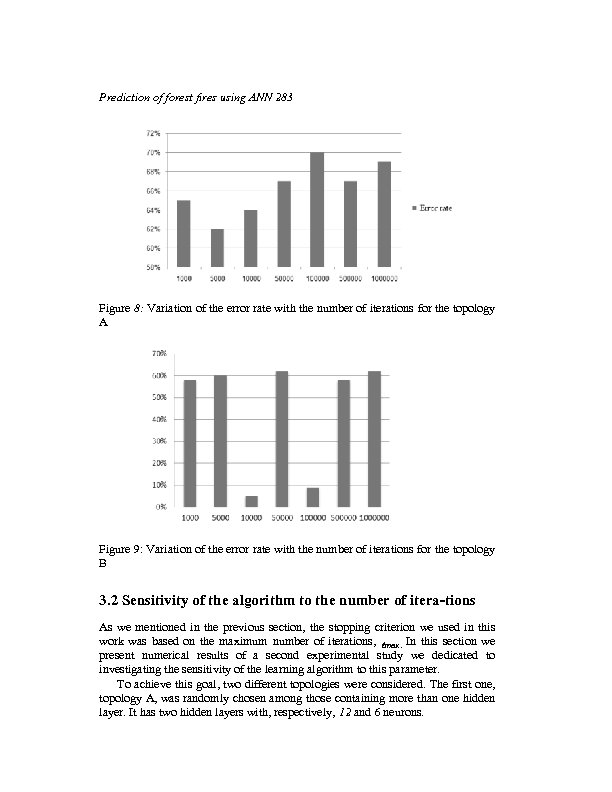Prediction of forest fires using ANN 283 Figure 8: Variation of the error rate with the number of iterations for the topology A Figure 9: Variation of the error rate with the number of iterations for the topology B 3. 2 Sensitivity of the algorithm to the number of itera-tions As we mentioned in the previous section, the stopping criterion we used in this work was based on the maximum number of iterations, tmax. In this section we present numerical results of a second experimental study we dedicated to investigating the sensitivity of the learning algorithm to this parameter. To achieve this goal, two different topologies were considered. The first one, topology A, was randomly chosen among those containing more than one hidden layer. It has two hidden layers with, respectively, 12 and 6 neurons.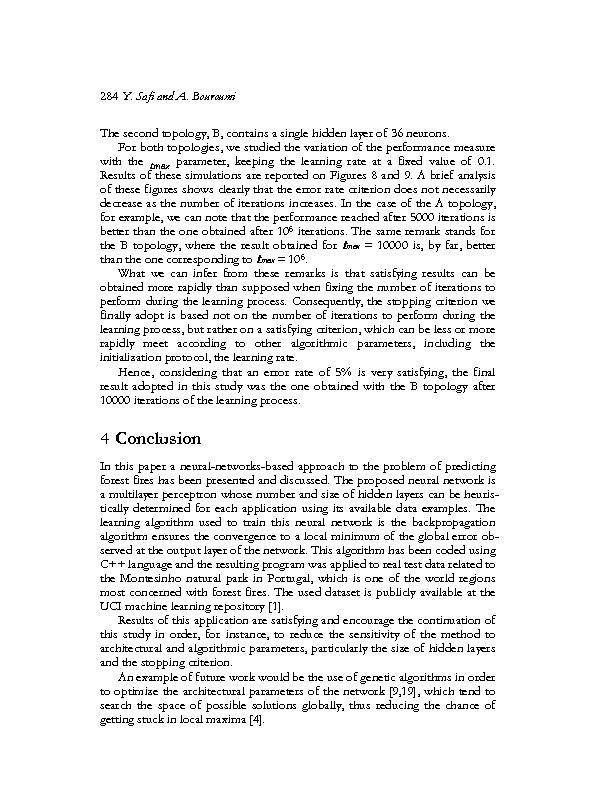284 Y. Safi and A. Bouroumi The second topology, B, contains a single hidden layer of 36 neurons. For both topologies, we studied the variation of the performance measure with the tmax parameter, keeping the learning rate at a fixed value of 0. 1. Results of these simulations are reported on Figures 8 and 9. A brief analysis of these figures shows clearly that the error rate criterion does not necessarily decrease as the number of iterations increases. In the case of the A topology, for example, we can note that the performance reached after 5000 iterations is better than the one obtained after 106 iterations. The same remark stands for the B topology, where the result obtained for tmax = 10000 is, by far, better than the one corresponding to tmax = 106. What we can infer from these remarks is that satisfying results can be obtained more rapidly than supposed when fixing the number of iterations to perform during the learning process. Consequently, the stopping criterion we finally adopt is based not on the number of iterations to perform during the learning process, but rather on a satisfying criterion, which can be less or more rapidly meet according to other algorithmic parameters, including the initialization protocol, the learning rate. Hence, considering that an error rate of 5% is very satisfying, the final result adopted in this study was the one obtained with the B topology after 10000 iterations of the learning process. 4 Conclusion In this paper a neural-networks-based approach to the problem of predicting forest fires has been presented and discussed. The proposed neural network is a multilayer perceptron whose number and size of hidden layers can be heuristically determined for each application using its available data examples. The learning algorithm used to train this neural network is the backpropagation algorithm ensures the convergence to a local minimum of the global error observed at the output layer of the network. This algorithm has been coded using C++ language and the resulting program was applied to real test data related to the Montesinho natural park in Portugal, which is one of the world regions most concerned with forest fires. The used dataset is publicly available at the UCI machine learning repository . Results of this application are satisfying and encourage the continuation of this study in order, for instance, to reduce the sensitivity of the method to architectural and algorithmic parameters, particularly the size of hidden layers and the stopping criterion. An example of future work would be the use of genetic algorithms in order to optimize the architectural parameters of the network [9, 19], which tend to search the space of possible solutions globally, thus reducing the chance of getting stuck in local maxima .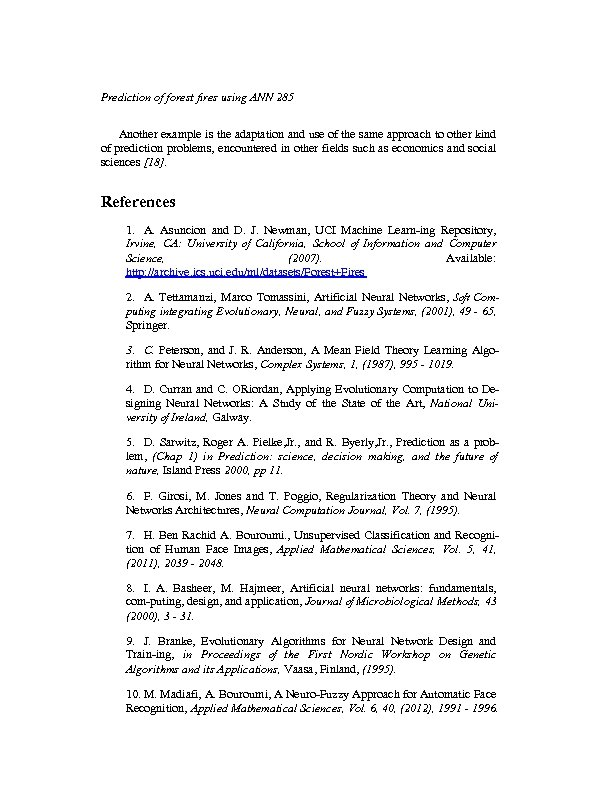Prediction of forest fires using ANN 285 Another example is the adaptation and use of the same approach to other kind of prediction problems, encountered in other fields such as economics and social sciences . References 1. A. Asuncion and D. J. Newman, UCI Machine Learn-ing Repository, Irvine, CA: University of California, School of Information and Computer Science, (2007). Available: http: //archive. ics. uci. edu/ml/datasets/Forest+Fires 2. A. Tettamanzi, Marco Tomassini, Artificial Neural Networks, Soft Computing integrating Evolutionary, Neural, and Fuzzy Systems, (2001), 49 - 65, Springer. 3. C. Peterson, and J. R. Anderson, A Mean Field Theory Learning Algorithm for Neural Networks, Complex Systems, 1, (1987), 995 - 1019. 4. D. Curran and C. ORiordan, Applying Evolutionary Computation to Designing Neural Networks: A Study of the State of the Art, National University of Ireland, Galway. 5. D. Sarwitz, Roger A. Pielke, Jr. , and R. Byerly, Jr. , Prediction as a problem, (Chap 1) in Prediction: science, decision making, and the future of nature, Island Press 2000, pp 11. 6. F. Girosi, M. Jones and T. Poggio, Regularization Theory and Neural Networks Architectures, Neural Computation Journal, Vol. 7, (1995). 7. H. Ben Rachid A. Bouroumi. , Unsupervised Classification and Recognition of Human Face Images, Applied Mathematical Sciences, Vol. 5, 41, (2011), 2039 - 2048. 8. I. A. Basheer, M. Hajmeer, Artificial neural networks: fundamentals, com-puting, design, and application, Journal of Microbiological Methods, 43 (2000), 3 - 31. 9. J. Branke, Evolutionary Algorithms for Neural Network Design and Train-ing, in Proceedings of the First Nordic Workshop on Genetic Algorithms and its Applications, Vaasa, Finland, (1995). 10. M. Madiafi, A. Bouroumi, A Neuro-Fuzzy Approach for Automatic Face Recognition, Applied Mathematical Sciences, Vol. 6, 40, (2012), 1991 - 1996.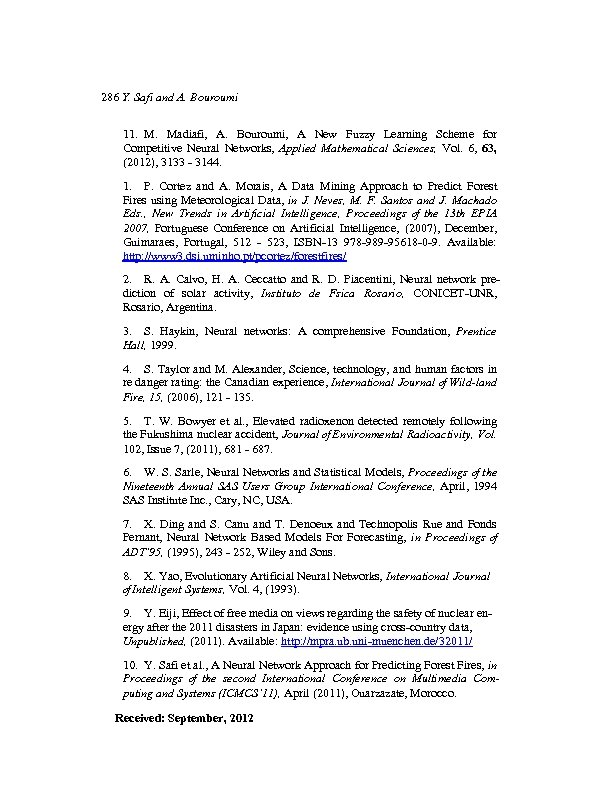286 Y. Safi and A. Bouroumi 11. M. Madiafi, A. Bouroumi, A New Fuzzy Learning Scheme for Competitive Neural Networks, Applied Mathematical Sciences, Vol. 6, 63, (2012), 3133 - 3144. 1. P. Cortez and A. Morais, A Data Mining Approach to Predict Forest Fires using Meteorological Data, in J. Neves, M. F. Santos and J. Machado Eds. , New Trends in Artificial Intelligence, Proceedings of the 13 th EPIA 2007, Portuguese Conference on Artificial Intelligence, (2007), December, Guimaraes, Portugal, 512 - 523, ISBN-13 978 -989 -95618 -0 -9. Available: http: //www 3. dsi. uminho. pt/pcortez/forestfires/ 2. R. A. Calvo, H. A. Ceccatto and R. D. Piacentini, Neural network prediction of solar activity, Instituto de Fsica Rosario, CONICET-UNR, Rosario, Argentina. 3. S. Haykin, Neural networks: A comprehensive Foundation, Prentice Hall, 1999. 4. S. Taylor and M. Alexander, Science, technology, and human factors in re danger rating: the Canadian experience, International Journal of Wild-land Fire, 15, (2006), 121 - 135. 5. T. W. Bowyer et al. , Elevated radioxenon detected remotely following the Fukushima nuclear accident, Journal of Environmental Radioactivity, Vol. 102, Issue 7, (2011), 681 - 687. 6. W. S. Sarle, Neural Networks and Statistical Models, Proceedings of the Nineteenth Annual SAS Users Group International Conference, April, 1994 SAS Institute Inc. , Cary, NC, USA. 7. X. Ding and S. Canu and T. Denoeux and Technopolis Rue and Fonds Pernant, Neural Network Based Models Forecasting, in Proceedings of ADT’ 95, (1995), 243 - 252, Wiley and Sons. 8. X. Yao, Evolutionary Artificial Neural Networks, International Journal of Intelligent Systems, Vol. 4, (1993). 9. Y. Eiji, Effect of free media on views regarding the safety of nuclear energy after the 2011 disasters in Japan: evidence using cross-country data, Unpublished, (2011). Available: http: //mpra. ub. uni-muenchen. de/32011/ 10. Y. Safi et al. , A Neural Network Approach for Predicting Forest Fires, in Proceedings of the second International Conference on Multimedia Computing and Systems (ICMCS’ 11), April (2011), Ouarzazate, Morocco. Received: September, 2012